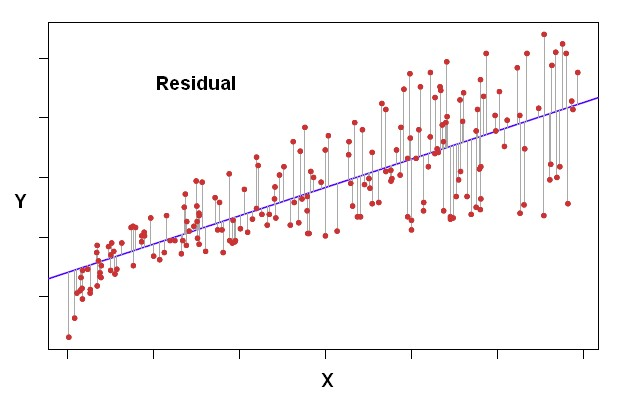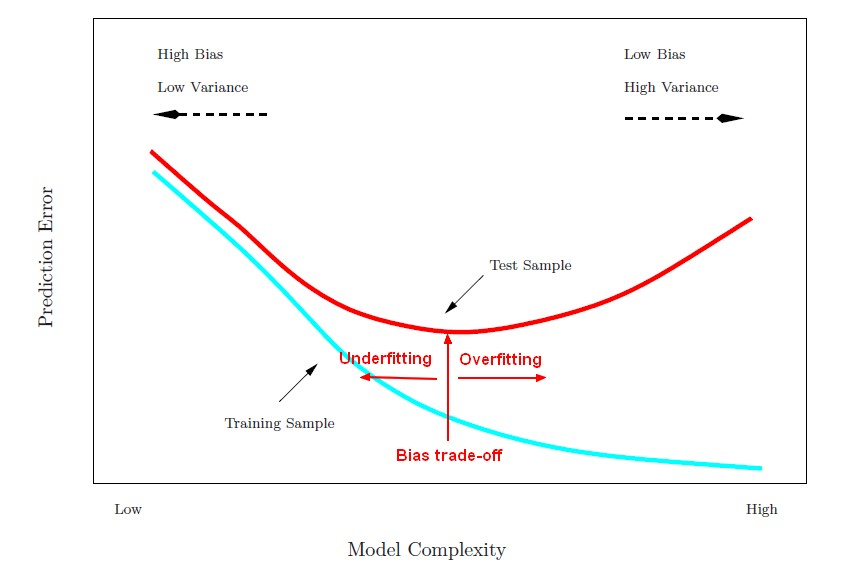# Statistics - Chance

The chance effect in Statistics is represented by the sampling error.

When something is unsystematic, it's then due to chance. If an error is systematic, it's a bias.

The chance probability is:

• 50% for a two binary event (tossing a coin).
• 33% for a three class classification

Chance is another word for random.

## Documentation / Reference

* If you’re so smart, why aren’t you rich? Turns out it’s just chance

Recommended PagesR - Logistic Regression

logistic regression in R We have a call to GLM where we gives: the direction: the response, and the predictors, the family equals binomial. This parameter tells GLM to fit a logistic regression...Statistics - (Confidence|likelihood) (Prediction probabilities|Probability classification)

Prediction probabilities are also known as: confidence (How confident can I be of this prediction?). or likelihood: (How likely is this prediction to be true?) They gives the probability of a predicted...Statistics - (Data Set|Sample)

Because of the difficulties of obtaining information all units in a population, it is common to use a small,random and representative subset of the population called a sample. A sample is a smaller,...Statistics - (F-Statistic|F-test|F-ratio)

The NHST anova statistic test is an F-test or F-ratio. It's what you observe in the numerator relative to what you would expect just due to chance in the denominator. The f statistic is the statistic...Statistics - (Residual|Error Term|Prediction error|Deviation) (e| )

The residual is a deviation score measure of prediction error in case of regression. The difference between an observed target and a predicted target in a regression analysis is known as the residual...Statistics - (dependent|paired sample) t-test

A dependent t-test is appropriate when: we have the same people measured twice. the same subject are been compared (ex: Pre/Post Design) or two samples are matched at the level of individual subjects...Statistics - (t-value|t-statistic)

The (t-value|t-statistic) is a test statistic. In NHST, it is essentially a ratio of what we observed relative to what we would expect just due to chance. Each t-value has corresponding p-value depending...Statistics - Bias-variance trade-off (between overfitting and underfitting)

The bias-variance trade-off is the point where we are adding just noise by adding model complexity (flexibility). The training error goes down as it has to, but the test error is starting to go up. The...Statistics - Null Hypothesis Significance Testing (NHST)

NHST is a procedure for testing the Null Hypothesis. It's a binary decision: Reject or Retain the Null Hypothesis Ie Retain the Null Hypothesis or the alternative hypothesis. Before starting any...Statistics - Power of a test

The power of a test sometimes, less formally, refers to the probability of rejecting the null when it is not correct, the chance that your experiment is right. A test's power is influenced by the choice...